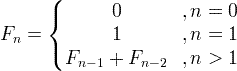# Fibonacci Numbers & Sequence

Fibonacci sequence is a sequence of numbers, where each number is the sum of the 2 previous numbers, except the first two numbers that are 0 and 1.

Contents

### Fibonacci sequence formulaFor example:

F0 = 0

F1 = 1

F2 = F1+F0 = 1+0  = 1

F3 = F2+F1 = 1+1  = 2

F4 = F3+F2 = 2+1  = 3

F5 = F4+F3 = 3+2  = 5

### Golden ratio convergence

The ratio of two sequential Fibonacci numbers, converges to the golden ratio:φ is the golden ratio = (1+√5) / 2 ≈ 1.61803399

TBD

### C code of Fibonacci function

 double Fibonacci(unsigned int n) {     double f_n =n;     double f_n1=0.0;     double f_n2=1.0;     if( n > 1 ) {         for(int k=2; k<=n; k++) {             f_n = f_n1 + f_n2;             f_n2 = f_n1;             f_n1 = f_n;         }     }     return f_n; }